# RS Aggarwal Class 6 Solutions Chapter 13 Angles and Their Measurement Ex 13A

## RS Aggarwal Class 6 Solutions Chapter 13 Angles and Their Measurement Ex 13A

These Solutions are part of RS Aggarwal Solutions Class 6. Here we have given RS Aggarwal Solutions Class 6 Chapter 13 Angles and Their Measurement Ex 13A.

Other Exercises

Question 1.
Solution:
Three examples are : Tongs, Scissors and Compasses.

Question 2.
Solution:
In the given angle ABC, the vertex is B and arms are $$\overrightarrow { AB }$$ and $$\overrightarrow { BC }$$ .

Question 3.
Solution:
(i) In the given figure, three angles are formed.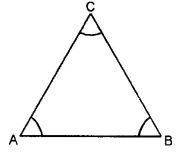Names of the angles are :
∠ABC, ∠BAC and ∠ACB
(ii) In the given figure, four angles are formed.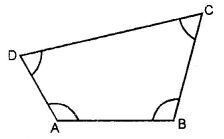(iii) In the given figure, eight angles are formed.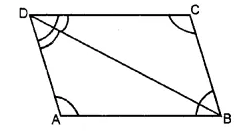Names of the angles are :

Question 4.
Solution:
In given figure
(i) Points S and Q are in the interior of ∠AOB
(ii) Points P and R are in the exterior of ∠AOB.
(iii) Points A, O, B, N, T lie on ∠AOB.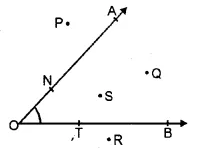Question 5.
Solution:
(i) False
(ii) True
(iii) False
(iv) True
(v) False

Question 6.
Solution:
In the given figure, another name for :
(i) ∠1 is ∠EPB
(ii) ∠2 is ∠PQC
(iii) ∠3 is FQD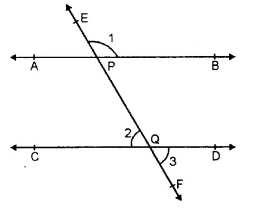Hope given RS Aggarwal Solutions Class 6 Chapter 13 Angles and Their Measurement Ex 13A are helpful to complete your math homework.

If you have any doubts, please comment below. Learn Insta try to provide online math tutoring for you.